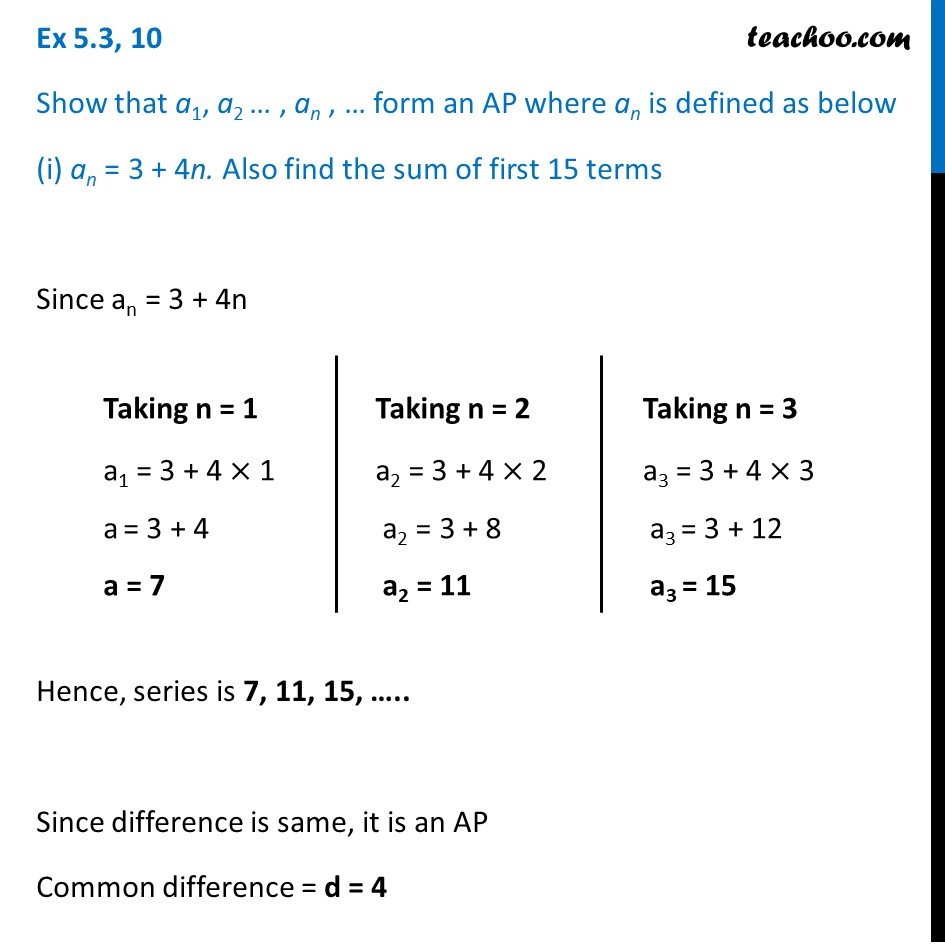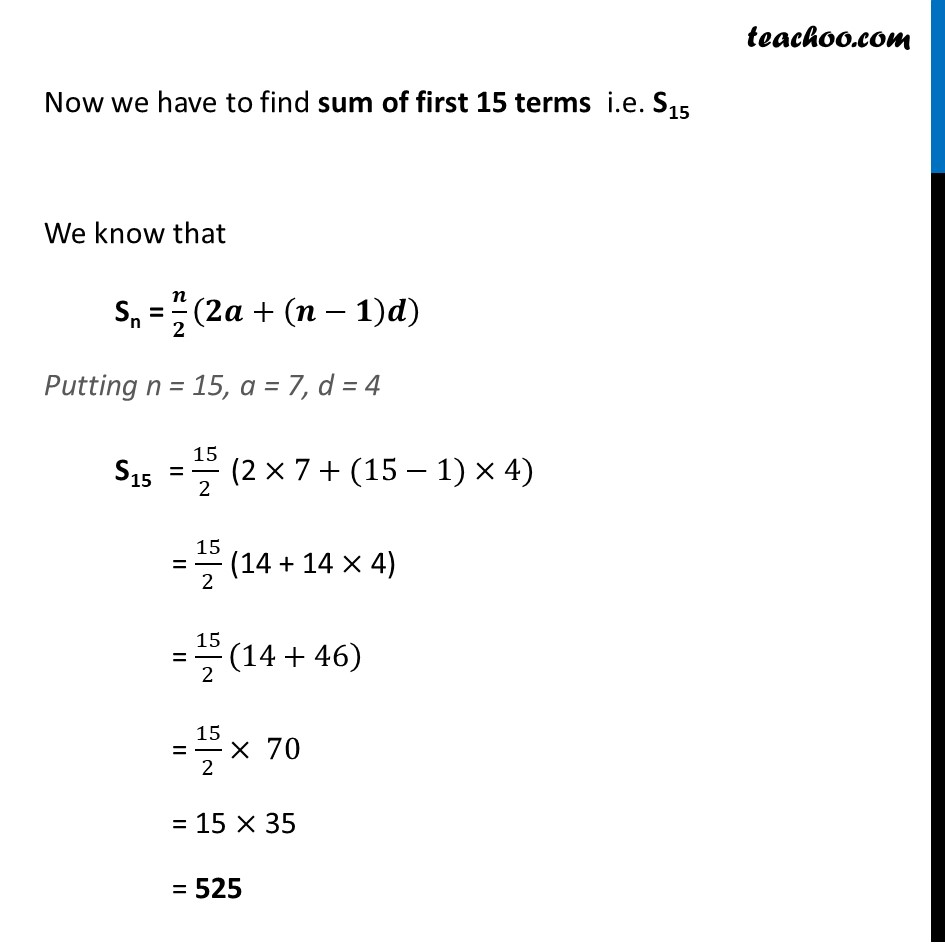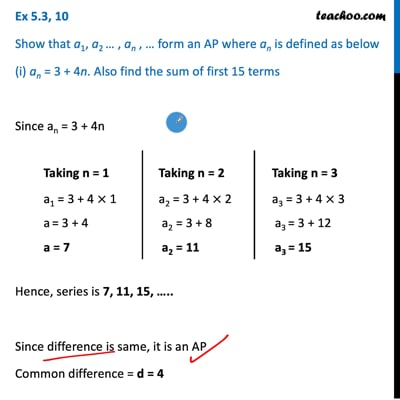Given nth term finding s

Chapter 5 Class 10 Arithmetic Progressions (Term 2)
Concept wiseThis video is only available for Teachoo black users

### Transcript

Ex 5.3, 10 Show that a1, a2 … , an , … form an AP where an is defined as below (i) an = 3 + 4n .Also find the sum of first 15 terms an = 3 + 4n Taking n = 1 a1 = 3 + 4 × 1 a = 3 + 4 a = 7 Taking n = 2 a2 = 3 + 4 × 2 a2 = 3 + 8 a2 = 11 Taking n = 3 a3 = 3 + 4 × 3 a3 = 3 + 12 a3 = 15 Now we have to find sum of first 15 terms i.e. S15 We know that Sn = 𝑛/2 (2𝑎+(𝑛−1)𝑑) Here, n = 15, a = 7, d = 4 Putting the values S15 = 15/2 (2 ×7+(15−1)×4) = 15/2 (14 + 14 × 4) = 15/2 (14+46) = 15/2×70 = 15 × 35 = 525 Hence series is 7, 11, 15, ….. Since difference is same, it is an AP Common difference = d = 4